# Division fractions - how to share fractions 🤔

## Concept of fraci

Fraction - One of the forms of representing a number in mathematics. This is a record in which a и bare numbers or expressions. There are two recording formats:

• Ordinary view - 1/2 or A / B,
• Decimal view - 0.5.

Above the line is taken to write divide, which is a numerator, and below the line is always a divider, which is called the denominator. The trait between the numerator and the denominator means division. In grade 5, the guys already know.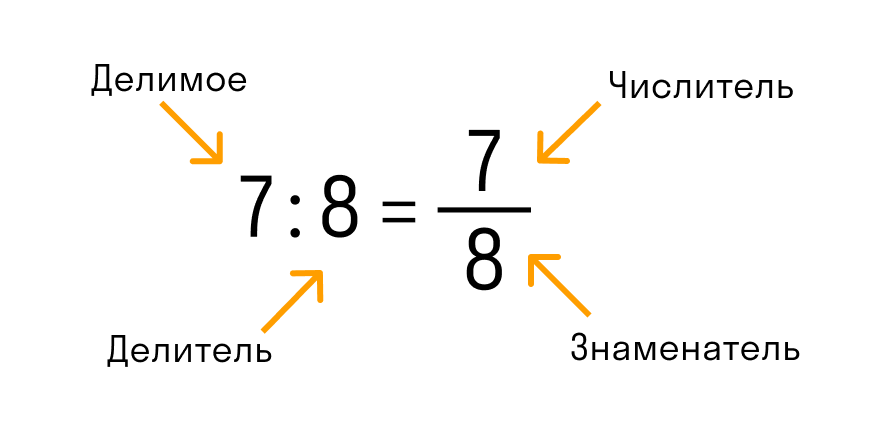The fraci is two types:

1. Numeric - consist of numbers, for example, 5/9 or (1.5 - 0.2) / 15.
2. Algebraic - consist of variables, for example, (x + y) / (x - y). In this case, the fraction value depends on these letter values.

Fraction is called correct When its numerator is less than the denominator. For example, 3/7 and 31/45.

Wrong - The one that has a numerator more denominator or equal to him. For example, 21/4. Such a number is mixed and read, as five as many as one fourth, and is recorded - 5 1 \ 4.

## The main properties of the fraci

1. The fraction does not matter, provided if the divider is zero.

2. The fraction is zero if the numerator is zero, and the denominator is different from zero.

3. Two fractions A / B and C / D are called equal, if A * d = b * c.

4. If the numerator and denominator multiply or divide on the same natural number, then the fraction equal to it.

## Division of fractional numbers

Division - Arithmetic action by which you can find out how many times one number is contained in the other. And the division is the opposite effect.

Properties of division:

1. When dividing per unit, the same number will be:

2. It is impossible to share for zero.

3. When we divide zero to any number, we always get zero:

4. When we divide any number on yourself we get a single one:

5. When we divide the amount for any number, you can divide each aligned to it, and then folded the resulting:

• (A + B): C = A: C + B: C.

6. When we divide the difference for some number, you can divide the reduced and subtractable separately and from the first private subtraction to the second:

• (A - B): C = A: C - B: C.

7. When we divide the work of two factors to the number, you can divide any of the multipliers and the private multiplied to the second factor:

• (A * B): C = (A: C) · B = A * (B: C).

## Division of ordinary fractions

How to share a fraction for a fraction? We carry out the following sequence of actions:

• The numerator is first multiplied by the denominator second, the result of the work is to write to the numerator of the new fraction;
• The denominator is the first to multiply on the numerator second, the result of the work is to write to the denominator of a new fraction.

In other words, this rule sounds like this: to divide one fraction to another, you must first multiply to the opposite one.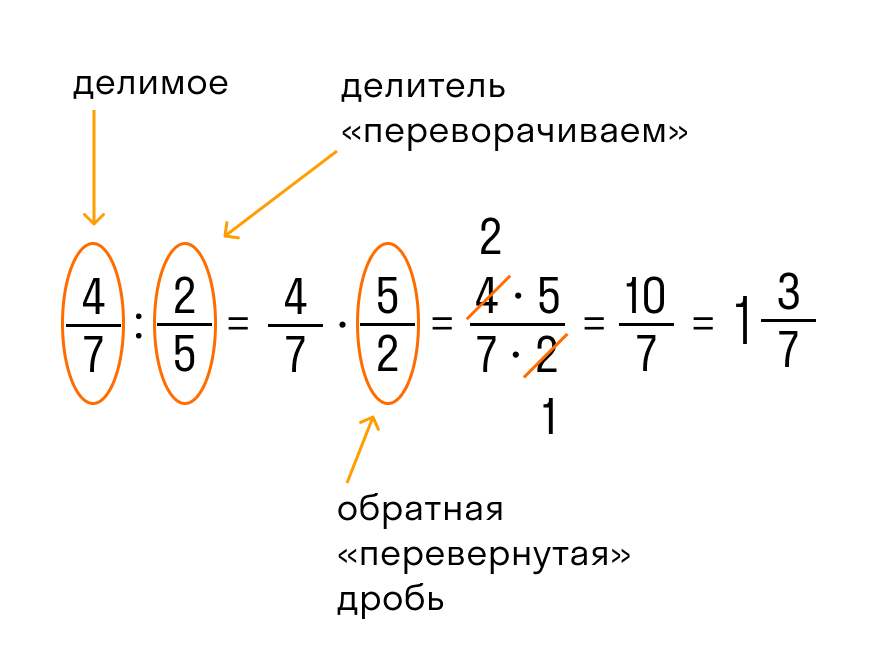How to share a fraction with different denominators? Everything is simple: we use the rules higher, because in practice it does not matter whether the same denominants or not.

## Dividing fractions on a natural number

For dividing the fraction on a natural number you need:

• submit this divider as an incorrect fraction, where the numerator is equal to this number, and the denominator unit;
• Decision by previous rules.## Dividing a natural number

To divide the natural number on an ordinary fraction you need:

• Denteller denominator multiplied by number;
• The divisor is recorded in the denominator.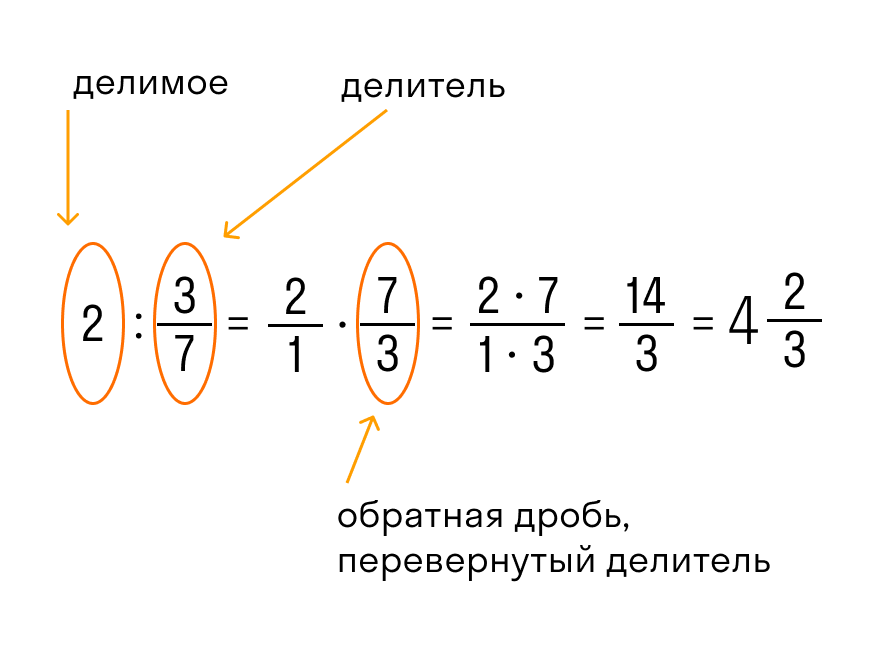## Mixed

For dividing mixed numbers, it is necessary:

• submit numbers in the form of incorrect fractions
• Divide what happened to each other.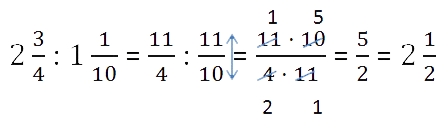If the lesson is in full swing and calculate you need to quickly - you can use the online calculator. Here are some suitable:

Come practice to Skysmart Children's School. Our teachers will understand anything - from fractions to sinus - and will answer questions that are awkward to set in front of the whole class. And also help catch up with peers and cope with complex control.

Instead of boring paragraphs, the child is waiting for interactive exercises with instant automatic check and online board, where you can draw and draw along with the teacher.

We will analyze how to split the number for the fraction, in theory and on specific examples.

To split the number on the fraction , need to:

1) a given number to multiply by the number, the opposite fraction (that is, the number is multiplied by an inverted fraction);

2) To multiply the number of fraction, it is necessary to multiply the numerator to this number, and the denominator is left for the same.

Examples .

Split the number for the fraction:To divide the number of fraction, this number must be multiplied by Number inverse This fraction (that is, the fraction turn over - the numerator and denominator change places).

Redfish 12 and 6 to 6. In the denominator received a unit, so the answer is an integer.When dividing the number to fraction the number rewrite and multiply on the fraction, the inverse one. Reducing 2 and 10 to 2.

Since they got the wrong fraction, it is necessary to highlight the whole part of it.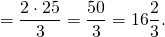To split the number on the fraction, dividable multiply by the number, reverse divider. We reduce 14 and 21 to 7. From the resulting incorrect fraction, we allocate the whole part.

Division of an integer

If an ordinary fraction is given, the division is performed as follows:

1) We find a fraction, inverse this ("turn over" it).

For example, 5/6 has a reverse fraction of 6/5, 2/3 has a back shot 3/2, etc.

2) Multiply the number on the resulting fraction.

When multiplying, the fluster is multiplied by an integer, and the denominator remains the same.Examples:

1) 6: (2/3) = 6 * (3/2) = 18/2 = 9.

2) 10: (5/4) = 10 * (4/5) = 8.

_

If a decimal fraction is given, it can be first present in the form of an ordinary fraction, and then perform division according to the rule that was given above.

Examples:

1) 5: 0.2 = 5: (2/10) = 5 * (10/2) = 25.

2) 12: 0.6 = 12: (6/10) = 12 * (10/6) = 20.

The next action that can be done with the fractions is division. Perform division fractions is quite simple to know several division rules. We will analyze the rules of division and consider the solution of examples on this topic.

## Decision fraction for fraction.

To share the fraction for the fraction, you need to fraction, which is a divider to flip, that is, to get a reverse fraction of the divider and then perform multiplication of fractions.

\ (\ bf \ frac {a} {b} \ div \ frac {c} {d} = \ frac {a} {b} \ Times \ FRAC {D} {C} \\\)

Example:

Perform the division of ordinary fractions.## Decision fracted by number.

To divide the fraction to the number, you need to multiply a denominator to the number.

\ (\ bf \ frac {a} {b} \ div n = \ frac {a} {b} \ div \ frac {n} {1} = \ frac {a} {b} \ Times \ FRAC {1} {n} \\\)

Consider an example:

Perform fractions on the natural number \ (\ FRAC {4} {7} \ div 3 \).

As we already know that any number can be represented as a fraction \ (3 = \ FRAC {3} {1} \).

\ (\ FRAC {4} {7} \ div 3 = \ frac {4} {7} \ div \ frac {3} {1} = \ FRAC {4} {7} \ Times \ FRAC {1} {3 } = \ FRAC {4 \ TIMES 1} {7 \ TIMES 3} = \ FRAC {4} {21} \\\)

## Division of the number of fraction.

To divide the number on the fraction, you need a valve denominator to multiply by the number, and the divisor numerator is written to the denominator. That is, the fraction of the divider turn over.

Consider an example:

Perform the division of the number to fraction.## Division of mixed frains.

Before proceeding with the division of mixed fractions, they need to be translated into the wrong fraction, and then the division according to the rules of fusion fraction.

Example:

Perform a division of mixed fractions.

\ (2 \ FRAC {3} {4} \ div 3 \ FRAC {1} {6} = \ FRAC {11} {4} \ div \ color {red} {\ FRAC {19} {6}} = \ FRAC {11} {4} \ Times \ Color {Red} {\ FRAC {6} {19}} = \ FRAC {11 \ Times 6} {4 \ Times 19} = \ FRAC {11 \ Times \ Color {Red } {2} \ Times 3} {2 \ Times \ Color {Red} {2} \ Times 19} = \ FRAC {33} {38} \\\)

## Division number by number.

To share simple numbers, you need to present them as a fraction and execute division according to the rules of fusion fraction for a fraction.

Example:

\ (2 \ div 5 = \ frac {2} {1} \ div \ color {red} {\ FRAC {5} {1}} = \ FRAC {2} {1} \ Times \ Color {Red} {\ FRAC {1} {5}} = \ FRAC {2 \ TIMES 1} {1 \ TIMES 5} = \ FRAC {2} {5} \\\)

Note to the topic division of fractions: it is impossible to divide to zero.

Questions on the topic: How to share a fraction? How to split fraction on the fraction? Answer: The fractions are divided into the same way, the first fraction is divisible multiplied by the fraction inversely fraction of the divider.

How to share a fraction with different denominators? Answer: It does not matter the same or different denominators in fractions, all the fractions are divided according to the rules of the fraction on the fraction.

Example number 1: Follow the division and name the divider, fraction, reverse divider: a) \ (\ FRAC {5} {9} \ div \ frac {8} {13} \) b) \ (2 \ FRAC {4} {5} \ Div 1 \ FRAC {7} {8} \)

Solution: a) \ (\ FRAC {5} {9} \ div \ frac {8} {13} = \ FRAC {5} {9} \ Times \ FRAC {13} {8} = \ FRAC {65} { 72} \\\\\)

\ (\ FRAC {8} {13} \) - divider, \ (\ FRAC {13} {8} \) - Reverse fraction of the divider.

b) \ (2 \ FRAC {4} {5} \ div 1 \ frac {7} {8} = \ FRAC {14} {5} \ div \ frac {15} {8} = \ FRAC {14} { 5} \ Times \ FRAC {8} {15} = \ FRAC {14 \ TIMES 8} {5 \ Times 15} = \ FRAC {112} {75} = 1 \ FRAC {37} {75} \\\\ \)

\ (\ FRAC {15} {8} \) - divider, \ (\ FRAC {8} {15} \) - Reverse fraction of the divider.

Example number 2: Calculate the division: a) \ (5 \ div 1 \ FRAC {1} {4} \) b) \ (9 \ FRAC {2} {3} \ div 8 \)

Decision:

a) \ (5 \ div 1 \ FRAC {1} {4} = \ FRAC {5} {1} \ div \ frac {5} {4} = \ FRAC {5} {1} \ Times \ FRAC {4 } {5} = \ FRAC {\ Color {Red} {5} \ Times 4} {1 \ Times \ Color {Red} {5}} = \ FRAC {4} {1} = 4 \\\\\)

b) \ (9 \ FRAC {2} {3} \ div 8 = \ FRAC {29} {3} \ div \ frac {8} {1} = \ FRAC {29} {3} \ Times \ FRAC {1 } {8} = \ FRAC {29 \ TIMES 1} {3 \ TIMES 8} = \ FRAC {29} {24} = 1 \ FRAC {5} {24} \\\\\)

Division fractions - how to share fractions 🤔